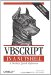# Using the Component

Once you've generated the .wsc file and written its code, there are two additional steps that may be required before you can use the component, depending precisely on how the component is to be used.

9.3.1 Registration

In most cases, unless the component is to be used exclusively to interface with DHTML in Microsoft Internet Explorer, it should be registered. The registration process stores information about the component that is needed to identify, locate, and activate it in the system registry. You can register your component in one of two ways:

• By right clicking on the file in Windows Explorer and selecting the Register option from the context menu.
• By typing the following from the command line:

`regsvr32 <componentFilename>`

where componentFilename is the name and extension of the .wsc file to be registered.

When registration has succeeded, a dialog appears that reads DllRegisterServer and DllInstall in <path>scrobj.dll succeeded.

9.3.2 Instantiating the Component

If you're using the component from VBScript, youinstantiate a script component like you would any other objectby calling the CreateObject function and passing it the programmatic identifier of the object to be created. You can then access the component's members. For instance, Example 9-3 shows a Windows Script Host script that instantiates the MathLib component and accesses each of its members. Programmatically, the scripted component is handled identically to a binary COM component.

Example 9-3. Using the MathLib component

```Dim math, sMsg
' Instantiate script component
Set math = CreateObject("MathLib.WSC")
WScript.ConnectObject math, "math_"

' Set and retrieve Value property
math.Value = 12.121
sMsg = "Value: " & math.Value & vbCrLf

sMsg = sMsg & "Pi: " & math.Pi & vbCrLf
sMsg = sMsg & "E:" & math.E & vbCrLf

' Call Min/Max methods
sMsg = sMsg & "Min: " & math.Min(10, 10.3) & vbCrLf
sMsg = sMsg & "Max: " & math.Max(1000,200) & vbCrLf

' Call Divide method
sMsg = sMsg & "Divide by 10: " & math.Divide(100, 10) & vbCrLf
sMsg = sMsg & "Divide by 0: " & math.Divide(2, 0) & vbCrLf

' Call IsEven/IsOdd methods
sMsg = sMsg & "Even: " & math.IsEven(12) & vbCrLf
sMsg = sMsg & "Even: " & math.IsEven(1) & vbCrLf
sMsg = sMsg & "Odd: " & math.IsOdd(12) & vbCrlf
sMsg = sMsg & "Odd: " & math.IsOdd(3) & vbCrlf

MsgBox sMsg

Public Sub math_DivByZero
Dim eMsg
eMsg = "Division by Zero Error " & vbCrLf & vbCrLf
eMsg = eMsg & Err.Number & ": " & Err.Description
eMsg = eMsg & Err.Source
MsgBox eMsg
End Sub```VBScript in a Nutshell, 2nd Edition
ISBN: 0596004885
EAN: 2147483647
Year: 2003
Pages: 335